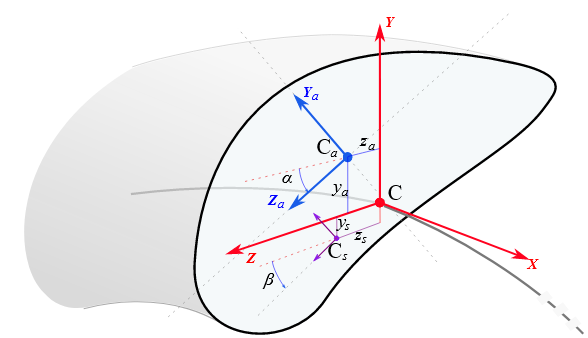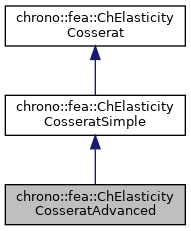## Description

Advanced linear elasticity for a Cosserat beam.

Uniform stiffness properties E,G are assumed through the section as in ChElasticityCosseratSimple, but here it also supports the advanced case of Iyy and Izz axes rotated respect reference, elastic center with offset from reference, and shear center with offset from reference. This material can be shared between multiple beams. The linear elasticity is uncoupled between shear terms S and axial terms A as to have this stiffness matrix pattern:

 n_x   [A       A A ]   e_x
n_y   [  S S S     ]   e_y
n_z = [  S S S     ] * e_z
m_x   [  S S S     ]   k_x
m_y   [A       A A ]   k_y
m_z   [A       A A ]   k_z#include <ChBeamSectionCosserat.h>[legend][legend]

## Public Member Functions

ChElasticityCosseratAdvanced (const double mIyy, const double mIzz, const double mJ, const double mG, const double mE, const double mA, const double mKs_y, const double mKs_z, const double malpha, const double mCy, const double mCz, const double mbeta, const double mSy, const double mSz)

void SetSectionRotation (double ma)
"Elastic reference": set alpha, the rotation of the section for which the Iyy Izz are defined, respect to the reference section coordinate system placed at centerline.

double GetSectionRotation ()

void SetCentroid (double my, double mz)
"Elastic reference": set the displacement of the elastic center (or tension center) respect to the reference section coordinate system placed at centerline.

double GetCentroidY ()

double GetCentroidZ ()

void SetShearRotation (double mb)
"Shear reference": set beta, the rotation of the section for shear decoupling, respect to the reference section coordinate system placed at centerline. More...

double GetShearRotation ()

void SetShearCenter (double my, double mz)
"Shear reference": set the displacement of the shear center S respect to the reference beam line placed at centerline. More...

double GetShearCenterY ()

double GetShearCenterZ ()

virtual void ComputeStress (ChVector<> &stress_n, ChVector<> &stress_m, const ChVector<> &strain_e, const ChVector<> &strain_k) override
Compute the generalized cut force and cut torque. More...

virtual void ComputeStiffnessMatrix (ChMatrixNM< double, 6, 6 > &K, const ChVector<> &strain_e, const ChVector<> &strain_k) override
Compute the 6x6 tangent material stiffness matrix [Km] = dσ/dε. More...Public Member Functions inherited from chrono::fea::ChElasticityCosseratSimple
ChElasticityCosseratSimple (const double mIyy, const double mIzz, const double mJ, const double mG, const double mE, const double mA, const double mKs_y, const double mKs_z)

void SetArea (double ma)
Set the A area of the beam.

double GetArea () const

void SetIyy (double ma)
Set the Iyy second moment of area of the beam (for bending about y in xz plane), defined as $$I_y = \int_\Omega z^2 dA$$. More...

double GetIyy () const

void SetIzz (double ma)
Set the Izz second moment of area of the beam (for bending about z in xy plane). More...

double GetIzz () const

void SetJ (double ma)
Set the J torsion constant of the beam (for torsion about x axis)

double GetJ () const

void SetKsy (double ma)
Set the Timoshenko shear coefficient Ks for y shear, usually about 0.8, (for elements that use this, ex. More...

double GetKsy () const

void SetKsz (double ma)
Set the Timoshenko shear coefficient Ks for z shear, usually about 0.8, (for elements that use this, ex. More...

double GetKsz () const

virtual void SetAsRectangularSection (double width_y, double width_z)
Shortcut: set Area, Ixx, Iyy, Ksy, Ksz and J torsion constant at once, given the y and z widths of the beam assumed with rectangular shape. More...

virtual void SetAsCircularSection (double diameter)
Shortcut: set Area, Ixx, Iyy, Ksy, Ksz and J torsion constant at once, given the diameter of the beam assumed with circular shape. More...

void SetYoungModulus (double mE)
Set E, the Young elastic modulus (N/m^2)

double GetYoungModulus () const

void SetGshearModulus (double mG)
Set G, the shear modulus.

double GetGshearModulus () const

void SetGwithPoissonRatio (double mpoisson)
Set G, the shear modulus, given current E and the specified Poisson ratio.

## Public Attributes

double alpha
Rotation of Izz Iyy respect to reference section, centered on line x.

double Cy
Elastic center, respect to reference section (elastic center, tension center)

double Cz

double beta
Rotation of shear reference section, centered on line x.

double Sy
Shear center, respect to reference section.

double SzPublic Attributes inherited from chrono::fea::ChElasticityCosseratSimple
double Iyy

double Izz

double J

double G

double E

double A

double Ks_y

double Ks_zPublic Attributes inherited from chrono::fea::ChElasticityCosserat
ChBeamSectionCosseratsection

## Constructor & Destructor Documentation

 chrono::fea::ChElasticityCosseratAdvanced::ChElasticityCosseratAdvanced ( const double mIyy, const double mIzz, const double mJ, const double mG, const double mE, const double mA, const double mKs_y, const double mKs_z, const double malpha, const double mCy, const double mCz, const double mbeta, const double mSy, const double mSz )
inline
Parameters
 mIyy Iyy second moment of area of the beam $$I_y = \int_\Omega z^2 dA$$ mIzz Izz second moment of area of the beam $$I_z = \int_\Omega y^2 dA$$ mJ torsion constant (torsion rigidity will be G*J, torsional stiffness = G*J*length) mG G shear modulus mE E young modulus mA A area mKs_y Timoshenko shear coefficient Ks for y shear mKs_z Timoshenko shear coefficient Ks for z shear malpha section rotation for which Iyy Izz are computed mCy Cy offset of elastic center about which Iyy Izz are computed mCz Cz offset of elastic center about which Iyy Izz are computed mbeta section rotation for which Ks_y Ks_z are computed mSy Sy offset of shear center mSz Sz offset of shear center

## ◆ ComputeStiffnessMatrix()

 void chrono::fea::ChElasticityCosseratAdvanced::ComputeStiffnessMatrix ( ChMatrixNM< double, 6, 6 > & K, const ChVector<> & strain_e, const ChVector<> & strain_k )
overridevirtual

Compute the 6x6 tangent material stiffness matrix [Km] = dσ/dε.

Parameters
 K 6x6 stiffness matrix strain_e local strain (deformation part): x= elongation, y and z are shear strain_k local strain (curvature part), x= torsion, y and z are line curvatures

Reimplemented from chrono::fea::ChElasticityCosseratSimple.

## ◆ ComputeStress()

 void chrono::fea::ChElasticityCosseratAdvanced::ComputeStress ( ChVector<> & stress_n, ChVector<> & stress_m, const ChVector<> & strain_e, const ChVector<> & strain_k )
overridevirtual

Compute the generalized cut force and cut torque.

Parameters
 stress_n local stress (generalized force), x component = traction along beam stress_m local stress (generalized torque), x component = torsion torque along beam strain_e local strain (deformation part): x= elongation, y and z are shear strain_k local strain (curvature part), x= torsion, y and z are line curvatures

Reimplemented from chrono::fea::ChElasticityCosseratSimple.

## ◆ SetShearCenter()

 void chrono::fea::ChElasticityCosseratAdvanced::SetShearCenter ( double my, double mz )
inline

"Shear reference": set the displacement of the shear center S respect to the reference beam line placed at centerline.

For shapes like rectangles, rotated rectangles, etc., it corresponds to the elastic center C, but for "L" shaped or "U" shaped beams this is not always true, and the shear center accounts for torsion effects when a shear force is applied.

## ◆ SetShearRotation()

 void chrono::fea::ChElasticityCosseratAdvanced::SetShearRotation ( double mb )
inline

"Shear reference": set beta, the rotation of the section for shear decoupling, respect to the reference section coordinate system placed at centerline.

The documentation for this class was generated from the following files:
• /builds/uwsbel/chrono/src/chrono/fea/ChBeamSectionCosserat.h
• /builds/uwsbel/chrono/src/chrono/fea/ChBeamSectionCosserat.cpp